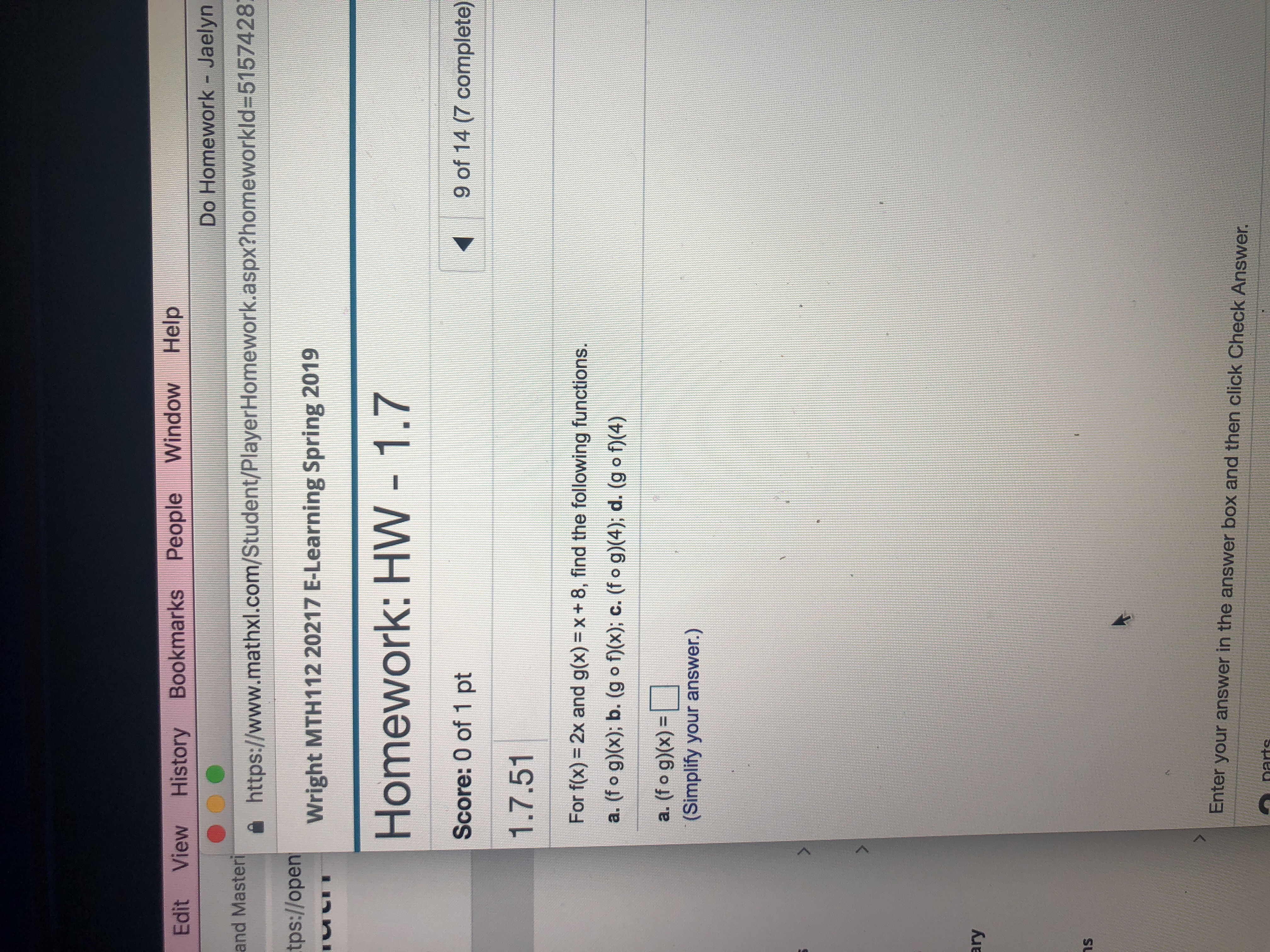# Edit View History Bookmarks People Window HelpDo Homework - Jaelynhttps://www.mathxl.com/Student/PlayerHomework.aspx?homeworkId=5157428wright MTH 112 20217 E-Learning Spring 2019Homework: HW - 1.7Score: 0 of 1 pt1.7.51tps://openuい·9 of 14 (7 complete)For f(x) = 2x and g(x)= x + 8, find the following functions.a. (f o g)x); b. (g o f)(x), c. (f o g) 4); d. (g o1(4)a(fo g))-(Simplify your answer.)arynsEnter your answer in the answer box and then click Check Answer

Question
4 viewshelp_outlineImage TranscriptioncloseEdit View History Bookmarks People Window Help Do Homework - Jaelyn https://www.mathxl.com/Student/PlayerHomework.aspx?homeworkId=5157428 wright MTH 112 20217 E-Learning Spring 2019 Homework: HW - 1.7 Score: 0 of 1 pt 1.7.51 tps://open uい· 9 of 14 (7 complete) For f(x) = 2x and g(x)= x + 8, find the following functions. a. (f o g)x); b. (g o f)(x), c. (f o g) 4); d. (g o1(4) a(fo g))- (Simplify your answer.) ary ns Enter your answer in the answer box and then click Check Answer fullscreen
check_circle

Step 1

a) (fog)(x)=f(g(x))= f(x+8) =2(x+8)=2x+16.

Step 2

b)(gof)(x)=g (f(x)) =g(2x)=2x+8.

Step 3

c)(fog)(4)=2(4...

### Want to see the full answer?

See Solution

#### Want to see this answer and more?

Solutions are written by subject experts who are available 24/7. Questions are typically answered within 1 hour.*

See Solution
*Response times may vary by subject and question.
Tagged in

### Functions